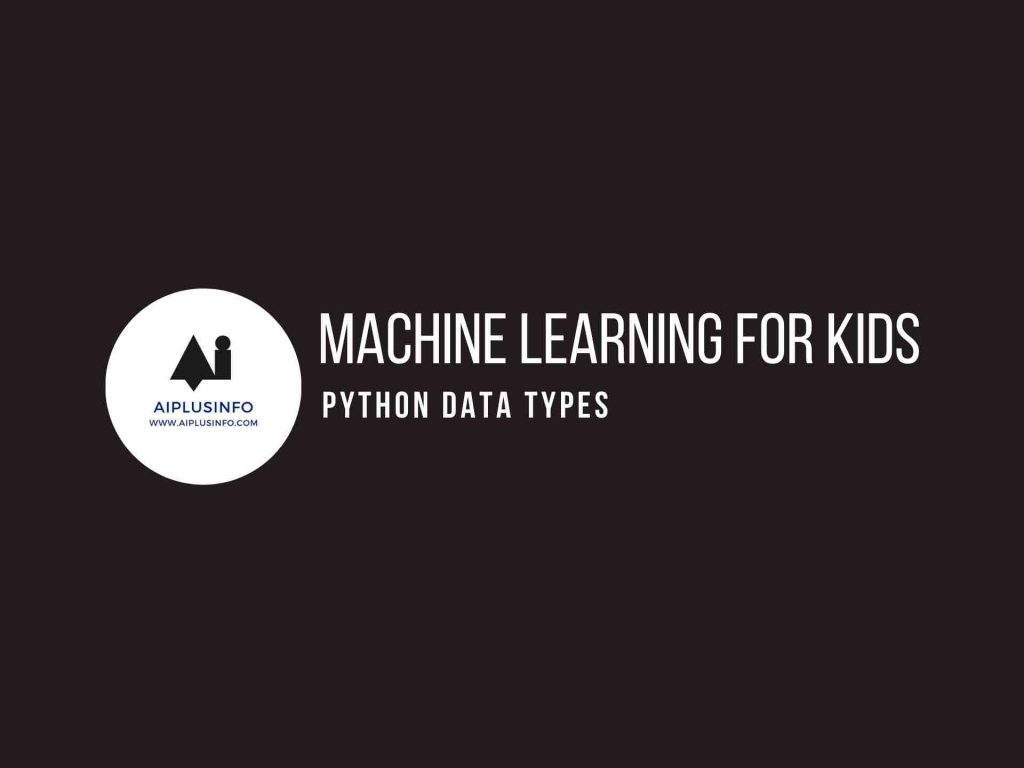# Machine Learning For Kids: Python Data Types## Introduction

Machine Learning for kids: Welcome to this tutorial on Python Data Types! Just like we have various types of data in real life such as numbers, text, lists of items, etc., Python also has several different types of data that it can recognize and manipulate. Understanding data types is a fundamental concept in programming.

## Who Is This For?

This tutorial is designed for young learners in grades 6 through 10. If you’ve been learning Python and are familiar with the concept of variables, then you’re ready to delve into the diverse world of Python data types!

## What Will We Learn?

In this journey of “Machine Learning for Kids” lets learn the basics of python which is the foundation of machine learning. In this tutorial, we will learn about the various types of data we can use in Python. These include numbers (both integers and decimals), text (called “strings” in Python), lists, and more. We will also learn how to determine the type of data stored in a variable.

## Understanding Python Data Types

Python recognizes several different types of data:

Numbers: These include integers (whole numbers) and floats (decimal numbers). For example, `5` is an integer and `7.3` is a float.

Strings: Strings are sequences of character data, essentially any text that you want to store. The string data type is represented by enclosing the text in quotes, like this: `"Hello, World!"`.

Lists: A list in Python is a collection of items which can be of mixed types. Lists are enclosed in square brackets `[...]` and the items are separated by commas. For example, `[1, "a", 4.5]` is a list containing an integer, a string, and a float.

Booleans: A Boolean data type has one of two possible values, `True` or `False`, which are generally used to represent the truth values of logical expressions.

Here is an example that demonstrates each of these types:

``````# Number - integer
my_int = 7
print(my_int)
print(type(my_int))

# Number - float
my_float = 7.3
print(my_float)
print(type(my_float))

# String
my_string = "Hello, World!"
print(my_string)
print(type(my_string))

# List
my_list = [1, "a", 4.5]
print(my_list)
print(type(my_list))

# Boolean
my_bool = True
print(my_bool)
print(type(my_bool))``````

In this code:

We assign different types of data to different variables.

We then use `print()` to print the value of each variable.

We also use the `type()` function, which tells us the data type of the variable.

The output of this code will be:

``````7
<class 'int'>
7.3
<class 'float'>
Hello, World!
<class 'str'>
[1, 'a', 4.5]
<class 'list'>
True
<class 'bool'>``````

As you can see, each type of data is recognized differently by Python, as indicated by the type names (‘int’, ‘float’, ‘str’, ‘list’, and ‘bool’). Understanding these different data types and how they behave is crucial as you learn to program in Python. In the following tutorials, we’ll learn more about how we can use and manipulate these data types. Happy coding!

## NEXT TUTORIALS

Tutorial 1 – Installing Python
Tutorial 2 – Your First Program in Python
Tutorial 3 – Python Variables
Tutorial 4 – Python Data Types
Tutorial 5 – Python Conditionals
Tutorial 6 – Python Loops
Tutorial 7 – Python Functions
Tutorial 8 – Advanced Python Functions
Tutorial 9 – Starter Machine Learning Python Program
Tutorial 10 – Your First Machine Learning Program!

Explore more with this book.

Python for Kids, 2nd Edition: A Playful Introduction to Programming
\$22.99
We earn a commission if you make a purchase, at no additional cost to you.
09/18/2023 12:47 am GMT

## References

Briggs, Jason R. Python for Kids: A Playful Introduction To Programming. No Starch Press, 2012.